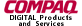glTranslate.3glOpenGL man pages

```

```

## Name

```  glTranslated,	glTranslatef - multiply	the current matrix by a	translation
matrix

```

## C Specification

```  void glTranslated( GLdouble x,
GLdouble y,
GLdouble z	)
void glTranslatef( GLfloat x,
GLfloat y,
GLfloat z )

```

## Parameters

```
x, y,	z
Specify the x,	y, and z coordinates of	a translation vector.

```

## Description

```  glTranslate moves the	coordinate system origin to the	point specified	by
(x,y,z).  The translation vector is used to compute a	4x4 translation
matrix:

| 1	   0	0    x	 |
|			 |
| 0	   1	0    y	 |
|			 |
| 0	   0	1    z	 |
|			 |
| 0	   0	0    1	 |

The current matrix (see glMatrixMode)	is multiplied by this translation
matrix, with the product replacing the current matrix.  That is, if M	is
the current matrix and T is the translation matrix, then M is	replaced with
M · T.

If the matrix	mode is	either GL_MODELVIEW or GL_PROJECTION, all objects
drawn	after glTranslate is called are	translated.  Use glPushMatrix and
glPopMatrix to save and restore the untranslated coordinate system.

```

## Errors

```  GL_INVALID_OPERATION is generated if glTranslate is executed between the
execution of glBegin and the corresponding execution of glEnd.

```

## Associated Gets

```  glGet	with argument GL_MATRIX_MODE
glGet	with argument GL_MODELVIEW_MATRIX
glGet	with argument GL_PROJECTION_MATRIX
glGet	with argument GL_TEXTURE_MATRIX

```

```  glMatrixMode,	glMultMatrix, glPushMatrix, glRotate, glScale